# Study on the Application of Model-based Control Algorithm for a Suspended Cable-Driven Parallel Robot

Title: Study on the Application of Model-based Control Algorithm for a Suspended Cable-Driven Parallel Robot

Corresponding email:

Pramujati, B., Syamlan, A.T., Nurahmi, L., Tamara, M.N., 2023. Study on the Application of Model-based Control Algorithm for a Suspended Cable-Driven Parallel Robot. International Journal of Technology. Volume 14(4), pp. 854-866

217
 Bambang Pramujati Department of Mechanical Engineering, Institut Teknologi Sepuluh Nopember, Surabaya, Indonesia, 60111 Adlina T. Syamlan Department of Mechanical Engineering, De Nayer Campus, Jan Pieter de Nayerlaan 5, 2860 Sint-Katelijne-Waver, KU Leuven, Belgium Latifah Nurahmi Department of Mechanical Engineering, Institut Teknologi Sepuluh Nopember, Surabaya, Indonesia, 60111 Mohamad Nasyir Tamara Department of Mechatronics Engineering, Electronic Engineering Polytechnic Institute of Surabaya, Surabaya, Indonesia, 60111
Email to Corresponding Author

Abstract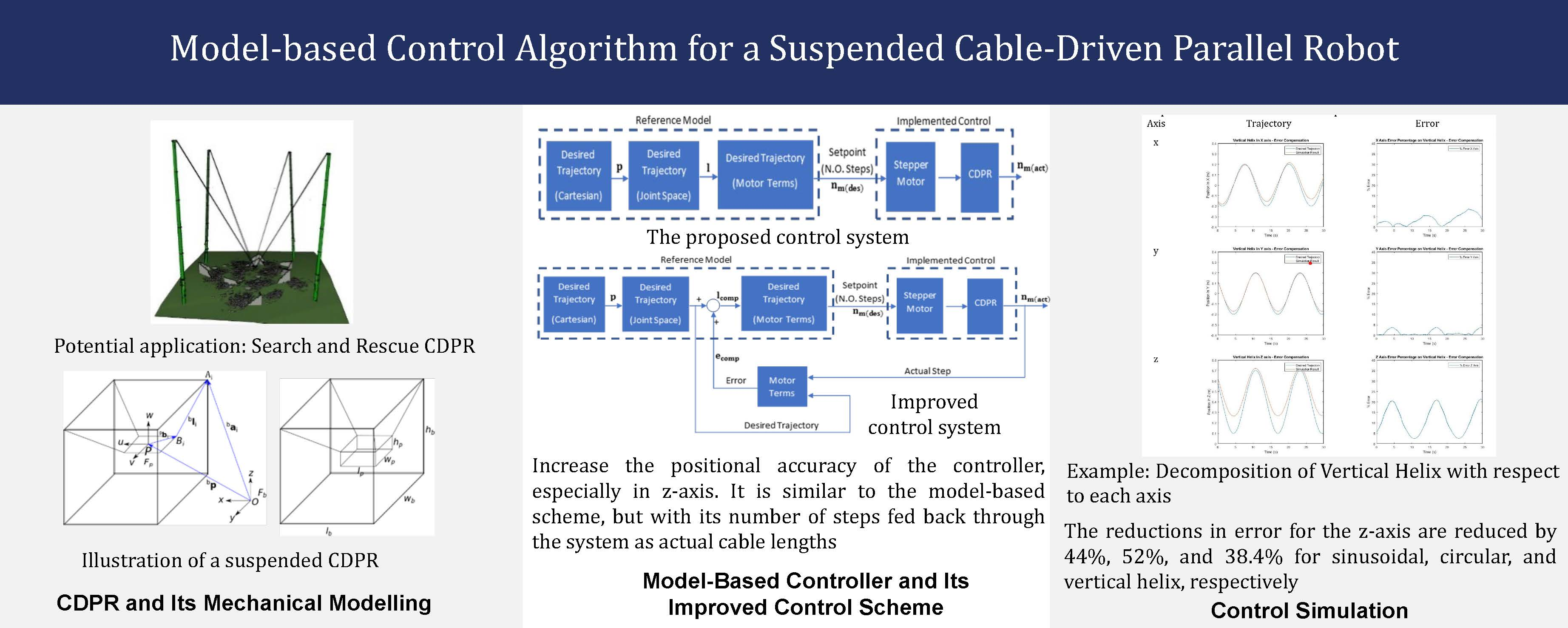This paper introduces a model-based control scheme for controlling the position of a suspended cable-driven parallel robot. The robot is designed to have a fixed frame base with four cables. The cables are attached to winches on one end, driven by stepper motors, and to a moving platform at the other end. The control scheme consists of two systems: the reference model and the implemented control. The implemented control hosts the stepper motor to drive the winch based on the requirements derived from the reference model. The reference model converts the desired Cartesian trajectory into joint spaces, which are then translated into the number of required steps. The number of steps will act as a set point for the stepper motor. Three trajectories are generated to test the compliance of the controller with its position. The error compensation scheme is introduced to increase the positional accuracy of the previous controller, especially on the z-axis. This algorithm uses the nature of discrete stepper motor movement to estimate the actual cable length, which is then fed back to the control system as an error. The control simulation results indicate a significant improvement in control performance, i.e. reduced position error, was achieved.

Cable-Driven Parallel Robot (CDPR); Error compensation; Model-based control; Stepper motor

Introduction

Nowadays, many different types of robots are used in industries, for example, arm and gantry robots. However, the use of these robots frequently comes with a number of issues related to their movement. (Baskoro, Kurniawan and Haikal, 2019). A new type of parallel manipulator that has emerged since the 1980s is a Cable-Driven Parallel Robot (CDPR). CDPR is a new type of manipulator where the rigid links are replaced by cables, giving it numerous advantages. Cables can bear a higher payload (Qian et al., 2018) due to their ability to withstand high tension. Unlike rigid links, cables can be actuated by coiling and uncoiling, which does not take up space, expanding their workspace (Gosselin, 2013). Moreover, cables have lower inertia and can be driven at high speeds (Qian et al., 2018). Capabilities possessed by CDPR have been realized in several industrial applications, such as material handling in port logistics (Holland and Cannon, 2003), aircraft maintenance (Nguyen and Goutterfarde, 2014), offshore sandblasting (Gagliardini et al., 2014), structural painting (Nguyen et al., 2014), large scale construction (Hussein, Santos, and Gouttefarde, 2018), rescue operation (Daney and Merlet, 2010), etc. CDPR can also be introduced as an alternative technology for search-and-rescue operations since it can cover a wide range of areas and has a high payload-to-weight ratio (Nurahmi et al., 2017).
Thus far, numerous topics related to CDPR, such as kinematics and path planning, have been extensively covered, but there is relatively limited information available on its control system. The control system of CDPR faces a significant challenge due to the flexible nature of cables, which can only exert force. Position of the moving platform is harder to control due to its flexibility, which leads to lower accuracy (Jung et al., 2016). The need to be in tension also implies that an under-constrained configuration cannot be fully controlled (Qian et al., 2018). A number of feedback control laws have been developed to tackle these issues, such as PD (Kawamura et al., 1995), Lyapunov-based and feedback linearization-based Proportional Derivative, PD (Alp and Agrawal, 2002), and Proportional Integral and Derivative, PID (Khosravi and Taghirad, 2014, Khosravi, Taghirad, and Oftadeh, 2013) to control the position of the end effector. However, the major drawback of these methods is that they do not consider the dynamics due to payload, thus leading to high position error. To solve this issue, more complex control has been introduced, such as sliding mode control (Hu et al., 2014), force control (Kraus et al., 2014), differential flatness (Yoon et al., 2018), and active stabilizer (Lesellier et al., 2018). Feed-forward compensators are also used for cable elasticity (Piao et al., 2017) and vibration reduction (Baklouti et al., 2019).

The main similarities of the aforementioned control schemes involve the use of sensors to read their set points. However, it is important to note that sensors are prone to noise, do not work effectively over long ranges, and require calibration. In addition, sensors can slow down assembly while effective disaster response is required to minimize financial losses brought on by disasters (Berawi et al., 2019). Since the search and rescue robot needs to be deployed quickly and easily, this will not work effectively. Therefore, technological innovation is crucial and required to meet these issues (Berawi, 2021). One of the alternatives to substitute the use of sensors is by utilizing a stepper motor as actuator. The Stepper motor moves in a discrete manner. This can be advantageous since it does not need a sensor to read the set point. The highest torque that a stepper motor can achieve is typically up to 50 Nm when using a NEMA 51 motor. This particular motor is capable of carrying a load of up to 500 kg.
In this paper, a model-based control algorithm will be presented for the suspended cable robot. Due to CDPR's appealing advantages, such as large workspace and high load capacity, relatively lightweight, low cost, etc., CDPR has gained large attention from many applications. In this paper, the illustration of the cable robot and its use to aid search and rescue operations are shown in Figure 1. The robot is designed to move debris due to natural disasters, and therefore, it does not need to be in high precision. The term model-based refers to the use of an actuator model in the control algorithm. A Stepper motor is used to drive the cables, with a number of steps taken as a set point. The discrete nature of moving in steps is used as feedback to the system. Therefore, the cable lengths can be calculated by using the linear-angular relationship between cables and the stepper motor. Three trajectories are selected based on past work carried out (Syamlan et al., 2019, Syamlan et al., 2020). The desired and actual trajectories will be compared along with their error, and the result will be used to suggest an improvement. The structure of this paper is as follows: The mechanical modeling of the cable robot and the motor is shown in section 2. Section 3 describes three potential work trajectories, and Section 4 describes the controller design, which contains the suggested and enhanced control systems. The simulation results for both control strategies on the specified trajectories are shown in Section 5's results and discussions.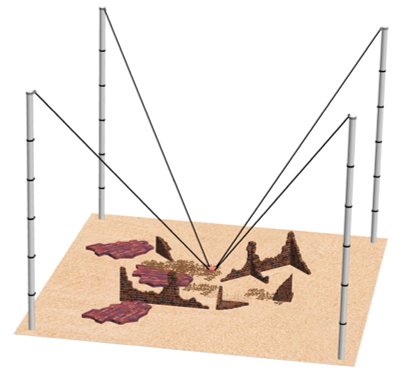Figure 1 Illustration of Search – and – Rescue CDPR

Experimental Methods

Mechanical Modelling

The reference model consists of the inverse geometric model and the motor model. It converts the desired trajectory from Cartesian space into joint space. The trajectory serves as input for geometric analysis, carried out to convert the desired position into the desired cable lengths. It is then translated into the number of steps that have to be generated by the stepper motor by using the motor model. In general, the reference model hosts mechanical modeling of the whole system, from the robot model to its supporting mechanisms. The geometric analysis and the motor model will be discussed briefly in this section.
2.1. Geometric and Dynamic Analyses
The suspended CDPR understudy comprises four cables, a cube moving platform, and a cube base frame, as shown in Figure 2. Each cable connects the cube-moving platform at the point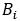to the base frame via a reconfigurable pulley at the point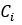, as shown in Figure 3. The moving platform is defined by length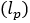, width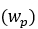, and height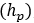, and the moving coordinate system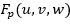. The geometric center P of the moving platform has its position coordinate expressed in the base frame denoted by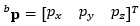. The origin of the base frame is denoted by point O. The base frame is situated on the bottom of the fixed frame, denoted by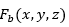. The base frame is defined by length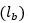, width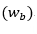, and height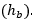. The pulley which can freely rotate about  axis of the angle  as depicted in Figure 3(b), is considered as a reconfiguration strategy. The pulley reconfiguration contributes to the robot motion and cable tension, as reported by (Syamlan et al., 2020).
The point in the moving platform needs to be expressed with respect to the base coordinate system by introducing rotation matrix R. Suppose the rotations about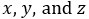axes are denoted by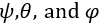. This is referred to as roll, pitch, and yaw, respectively. Then, the orientation of the moving frame with respect to the base frame can be expressed as: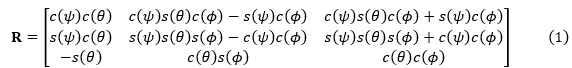Combining both the translational and rotational positions of the moving platform become: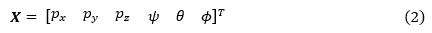Each cable runs through a pulley, connecting the moving platform to the fixed frame. The cable end that is attached to the moving platform is referred to as anchor points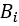, where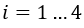. The position vector of these anchor points is expressed with respect to the platform frame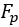, denoted as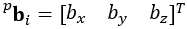. Pulleys are attached to each side on the top of the fixed frame. The cable enters the pulley at the contact point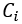and exits at the point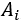, as shown in Figure 3(a).  The position vector of these contact points is expressed with respect to the base frame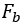, denoted as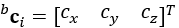. The cable distance between the contact point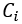and anchor point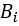is the cable length, denoted as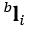. Therefore, by using loop closure, the vector of cable lengths can be determined as follows: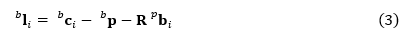For more detailed mathematical expressions of the reconfigurable pulley at the contact point, the readers may refer to (Syamlan et al., 2020).
The unit vector of cables is derived as: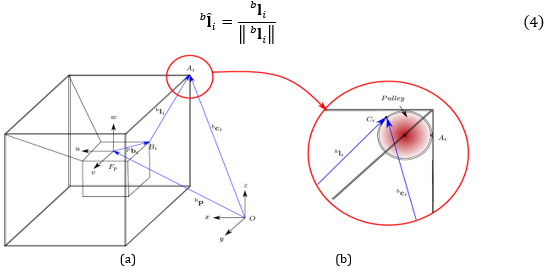Figure 2 Geometric representation of a suspended CDPR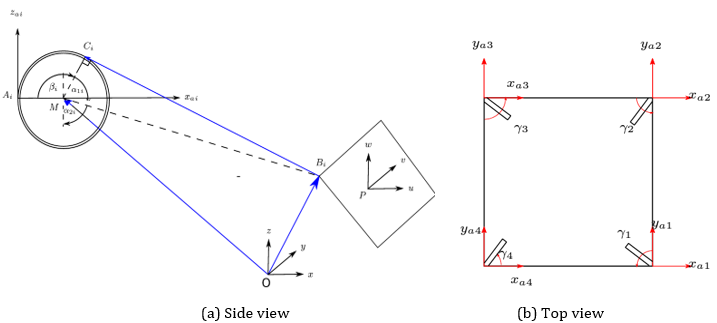Figure 3 Detailed description of a pulley mechanism
The cable mass and stiffness are negligible since the cable is relatively lighter than the rigid links. The unit vector of the cable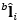is used to determine the wrench matrix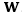. This matrix is useful to derive the dynamics of CDPR based on the Newton Law of motion, as follows: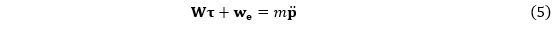Where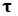is the cable tension,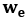is the external wrench,  is the mass of the moving platform, and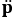is the acceleration of the moving platform. The trajectory generation discussed hereafter will provide the information corresponding to the position, velocity, and acceleration of the moving platform. Given the acceleration, the cable tension can be deduced from Equation (5). Since each cable is mounted to the winch and motor, the cable tension from Equation (5) can be used directly to compute the motor torque depending on the motor shaft diameter.

2.2. Motor Modelling

The cable lengths obtained from the geometric model need to be represented in terms of its actuators. In this case, stepper motors are used to drive the cables. Stepper motors are chosen because they move by number of steps, which is the multiplication of their step angle. Therefore, actual steps can be gathered without the need for an additional sensor. Before deriving this relationship, some assumptions are taken into account as follows:

1. Pulley and cables are assumed mass-less and friction between pulley and cables is assumed negligible.

2. Winches are assumed to always coil only one layer of cable.

3. Transmission loss within the actuator is assumed negligible.

4. No missed steps were generated by the stepper motor.

The current control scheme applied to the robot focuses solely on position control. The rotation of the shaft angle acts as the set point to the control scheme. The stepper motor moves the shaft to the desired angle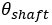determined by the number of steps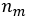that must be generated according to its step angle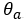, and mathematically can be expressed as: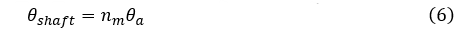The relationship between the desired cable length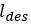and the desired shaft angle can be written as: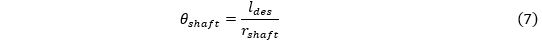where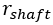defined the shaft radius. Hence, the number of steps required to generate the desired shaft angle based on the desired cable length can be mathematically expressed as: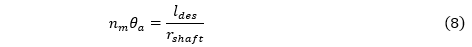Rearranging the equation to find a number of steps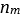gives: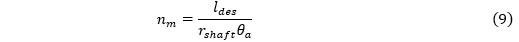Trajectory Generation

Three trajectories, namely sinusoidal, circular, and vertical helix trajectories, are generated in this paper based on (Gosselin, 2010). The design parameters of the CDPR model shown in Table 1 are referenced from previous work on the suspended CDPR conducted by (Syamlan, 2020). The results corresponding to the platform position between the desired values versus the actual one and its error will be presented for each trajectory.

Table 1 Design Parameters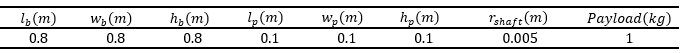3.1. Sinusoidal Trajectory
The moving platform following the sinusoidal trajectory oscillates in the -axis. The moving platform position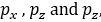expressed with respect to the base frame, has its oscillation frequency and amplitude of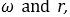respectively. The initial height of the platform is denoted as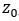, which in this case is set to be equal to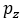. The position can be expressed as: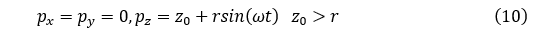The velocities and accelerations are obtained by deriving Equation (10) with respect to time. The trajectory parameters for the sinusoidal wave used in this paper are based on (Syamlan, 2020), as shown in Table 2.
Table 2 Trajectory Parameters for the Sinusoidal Motion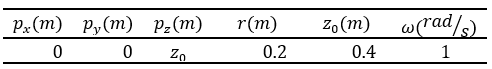3.2. Circular Trajectory
The second trajectory generated in this paper is a circular trajectory lying on the xy-plane. The position of the moving platform tracing this trajectory is expressed with respect to the base frame. The initial height of the moving platform is denoted by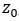. The rotating frequency and amplitude of the trajectory are defined as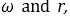respectively. The moving platform poses tracing this trajectory can be mathematically determined as: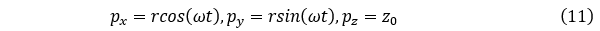By deriving Equation (11) with respect to time, the velocities and accelerations of the moving platform are determined. The parameters that are used to construct the circular trajectory are based on (Syamlan, 2020), as shown in Table 3.
Table 3 Trajectory Parameters for the Circular Trajectory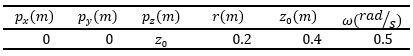3.3. Vertical Helix
The vertical helix trajectory is a more complex. The platform rotates with respect to the -axis with an amplitude of  while simultaneously performing an oscillation in the vertical direction. The frequencies for both the rotation and oscillation are denoted by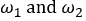respectively. The initial height of the platform is set as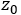, which is equal to . The amplitude of oscillation is denoted by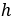. The moving platform position executing the vertical helix trajectory can be mathematically defined as: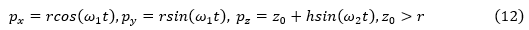By performing the time derivative for Equation (12), the velocities and accelerations of point P can be defined. The values assigned to each parameter are based on (Syamlan, 2020), as summarized in Table 4.
Table 4 Trajectory Parameters for the Vertical Helix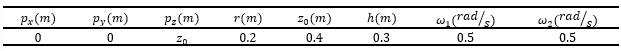Controller Design
4.1. Proposed Control Scheme
The proposed control scheme comprised two sub-systems, a reference model and an implemented control. The reference model generates set points for the controller. It converts Cartesian trajectory into the required cable lengths for each motor. These values are then translated into equivalent shaft angles. A number of steps obtained from the shaft angle will be used as a set point for the Implemented control. The proposed block diagram of the system is shown in Figure 4.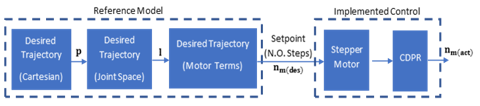Figure 4 Block Diagram of the Proposed Control Scheme.
Each cable will be driven by a Sumtor 57HS6425A4D8 stepper motor. The specification of the stepper motor is shown in Table 5.
Table 5 Motor Specification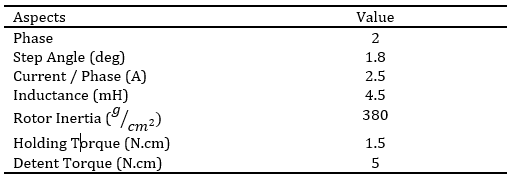4.2.  Improved Control Scheme
The proposed control scheme is enhanced by the error compensation scheme. This control strategy was created to improve the controller's position precision, particularly on the z-axis. It is analogous to the model-based scheme, with its number of steps being fed back through the system as an actual cable length, as shown in Figure 5.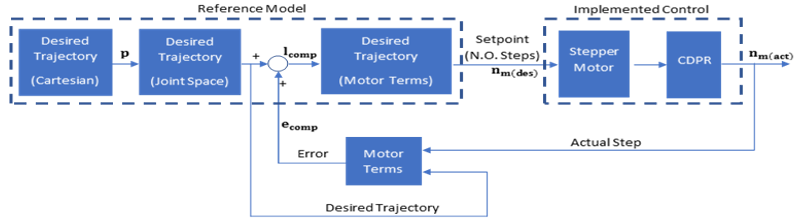Figure 5 Block Diagram of Improved Control Scheme.
Multiplying the number of steps by the shaft radius will give the actual cable length as follows: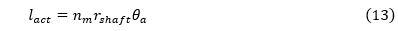Routing this value back and subtracting this value from the desired cable length will give a value that is like a position error. The error will be added to the system as an addition to determine the compensated length as follows: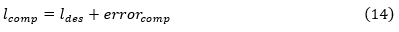The difference between the actual and desired cable length is defined as error comp, which can be mathematically expressed as follows: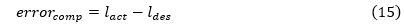This improved control scheme is applied to the CDPR understudy such that the motion of the moving platform will follow as close as possible to the generated trajectory. The results are presented and discussed hereafter.

Results and Discussion

The comparisons between the actual and desired position of the moving platform when tracing the sinusoidal wave by using model-based control are shown in Figure 6(a).  There is a considerable difference between the set point and the actual position when performing a downward motion. The observable error on the bottom of each valley of the sinusoidal wave is as high as 0.2 m, which resulted in an error of 26% on average. The motion is then improved by applying the error compensation control, and the results between the actual and desired pose of the moving platform are shown in Figure 6(b). When executing the sinusoidal wave, the robot demonstrates improved position accuracy, resulting in a notable reduction of overshoot. On average, the position error is reduced by 44%, decreasing from 26% to 14.5%, as illustrated in Figure 7(a)-(b). The rise in error to 22.6% on the first peak is due to the initial setup of the actuators matching the setpoint.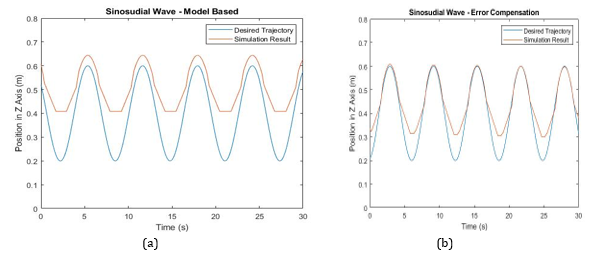Figure 6 Results from Sinusoidal Motion (a) Model-based control, (b) Error compensation control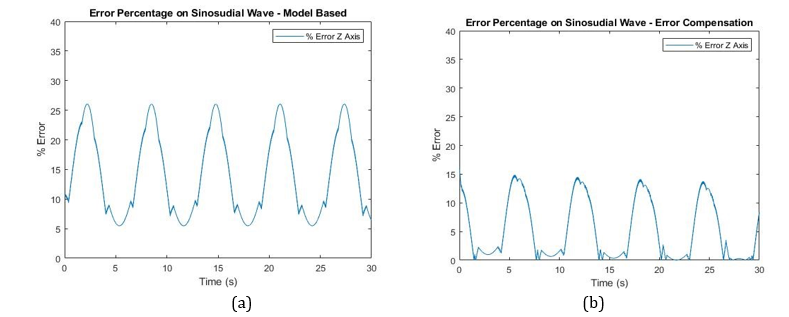Figure 7 Errors from Sinusoidal Motion (a) Model-based control, (b) Error compensation control.

The comparisons between the actual and desired circular trajectory by using a model-based control scheme are shown in Figure 8(a). Notice that the actual trajectory has a smaller radius and higher position than the desired trajectory. Its pose breakdown with respect to each axis is summarized in Table 6. It is observed that the difference in its circular radius for both the x and y axis is at 0 .023 m. The highest error for each axis is at 9.5% and 14.2%, respectively. There is a significant gap between the desired and actual pose in z-axis of 0.12 m, which resulted in an error of 14 .9%. The motion is greatly improved when the error-compensation control is applied, as shown in Figure 8(b). The moving platform is now able to follow the desired pose in terms of its circular radius if compared to the previous control scheme. The pose breakdown with respect to each axis is summarized in Table 6. By ignoring the initial setup, improvements are seen for all axis and are noticeable, especially in -axis. Both the motions about  and  axes have a considerable improvement in compliance, with a reduction in error of 50.5% and 62% to 5.3% and 5.7%, respectively. In terms of -axis, the actual pose is significantly lower than before, with a 52% error reduction to 7.2% on average.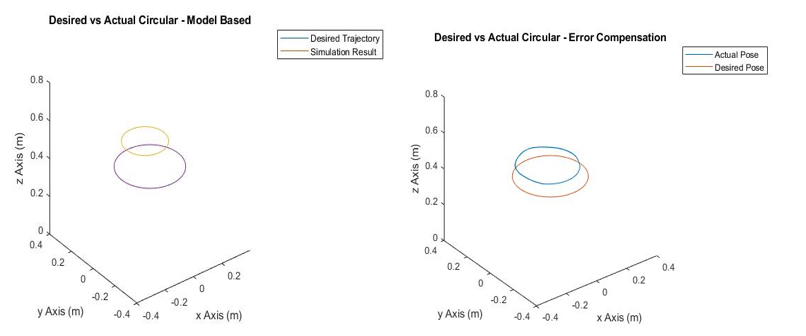Figure 8 Results from Circular Horizontal Motion (a) Model-based control, (b) Error compensation control.

Table 6 Decomposition of Circular Trajectory with respect to each axis.